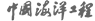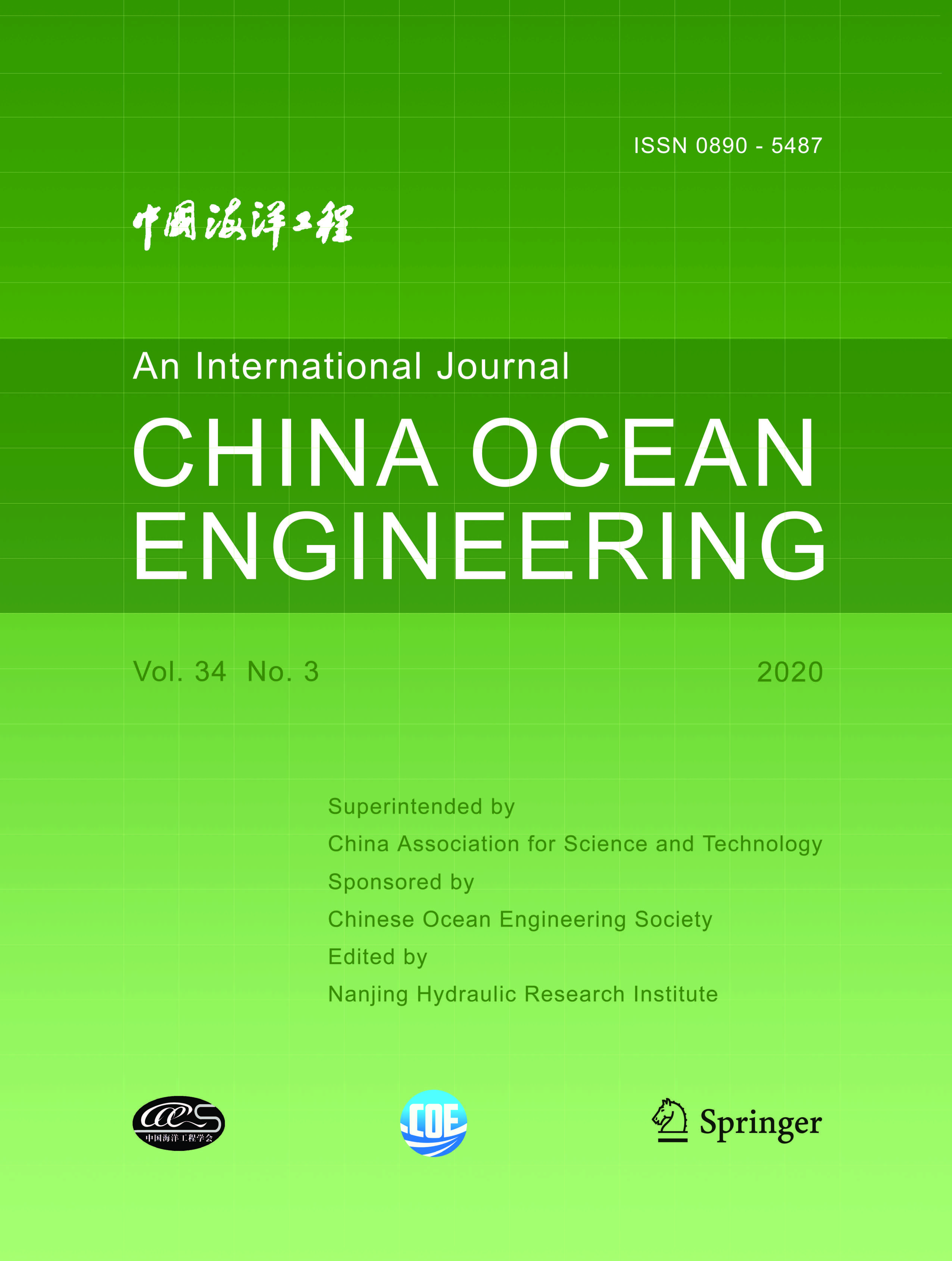ISSN  0890-5487 CN 32-1441/P

## 2000 Vol.(1)

Display Mode：          |

2000, (1): -.
[Abstract](510)
Abstract:
In this paper, integrating the Yangtze Estuary with the Hangzhou Bay, a 2-D velocity field model is established. In the model, fine self-adaptive grids are employed to adapt to the complicated coastal shape. The hydrodynamic equations satisfied by two contravariant components of velocity vector and surface elevation in non-orthogonal curvilinear coordinates are used. In each momentum equation the coefficients before the two partial derivatives of surface elevation with respect to variables of alternative direction coordinates have different orders of magnitude, i. e., the derivative with the larger coefficient may play a more important role than that with the smaller one. With this advantage, the ADI scheme can be easily employed. The hydrodynamic factors include tidal current, river runoff and wind-induced current. In terms of tidal current, seven main constituents in the area are considered in the open boundaries. The verifications of surface elevation process and current velocity process in the spring tide and in the neap tide show that the model can preferably reflect current fields in the area. Through the simulation of Lagrangian residual current fields in summer and in winter, the paths of the exchange of water and sediment between the Yangtze Estuary and the Hangzhou Bay are elementarily discussed.
2000, (1): -.
[Abstract](336)
Abstract:
Presented is the numerical analysis of settlements of soft soil by a 2-D dynamic effective stress FEM method. The model based on the results of cyclic triaxial tests on the reconstituted soft Ariake clay is used to predict the wave induced excess pore water pressure and residual strain of soft clay. The settlements of two types of breakwaters on the soft clay under ocean wave load, a low embankment subjected to traffic load and the tunnel surrounded by soft clay in Shanghai subjected to locomotive load are calculated as examples.
2000, (1): -.
[Abstract](338)
Abstract:
Traditionally, the use of a tuned mass damper (TMD) is to improve the surviability of the primary structure under extraordinary loading environment while the design loading condition is described by either a harmonic function or a stationary random process that can be fully characterized by a power spectral density (PSD) function. Aiming at prolonging the fatigue life of an offshore platform, this study considers an optimal design of TMD for the platform under long- term nonstationary loading due to long-term random sea waves characterized by a probabilistic power spectral density (PPSD) function. In principle, a PPSD could be derived based on numerous ordinary PSD functions; and each of them is treated as realization of the corresponding PPSD. This study provides a theoretical development for the optimal TMD design by minimizing the cost function to be the mean square value of the expected long-term response. A numerical example is presented to illustrate the developed design procedure.
2000, (1): -.
[Abstract](340)
Abstract:
In Bohai Gulf, offshore and other installations have collapsed by sea ice due to the fatigue and fracture of the main supporting components in the ice environments. In this paper presented are some results on fatigue reliability of these structures in the Gulf by investigating the distributions of ice parameters such as its floating direction and speed, sheet thickness, compressive strength, ice forces on the structures, and hot spot stress in the structure. The low temperature, ice breaking modes and component fatigue failure modes are also taken into account in the analysis of the fatigue reliability of the offshore structures experiencing both random ice loading and low temperatures. The results could be applied to the design and operation of offshore platforms in the Bohai Gulf.
2000, (1): -.
[Abstract](324)
Abstract:
2000, (1): -.
[Abstract](322)
Abstract:
2000, (1): -.
[Abstract](332)
Abstract:
2000, (1): -.
[Abstract](331)
Abstract:
As a further development of the authors' work (Huang and Qian, 1993), in this paper a new numerical method based on the time domain boundary element technique is proposed for solving fluid-solid coupling problems, in which a rigid body impacts normally on the calm surface of a half-space fluid. A fundamental solution to the half-space potential flow problem is first derived with the method of images. Then, an equivalent boundary integral equation in the Laplace transform domain is established by means of Green's second identity. Through the inverse Laplace transform and discretization in both time and boundary of the fluid region, the numerical calculation for the problem under consideration has been carried out. Several examples demonstrate that the present method is more efficient than existing ones, from which it is also seen that the shape of the impacting body has a considerable effect on the total impact force.
2000, (1): -.
[Abstract](319)
Abstract:
2000, (1): -.
[Abstract](338)
Abstract:
An experimental study of regular wave and irregular wave breaking is performed on a gentle slope of 1:200. In the experiment, asymmetry of wave profile is analyzed to determine its effect on wave breaker indices and to explain the difference between Goda and Nelson about the breaker indices of regular waves on very mild slopes. The study shows that the breaker index of irregular waves is under less influence of bottom slope i, relative water depth d/ L0 and the asymmetry of wave profile than that of regular waves. The breaker index of regular waves from Goda may be used in the case of irregular waves, while the coefficient A should be 0.15. The ratio of irregular wavelength to the length calculated by linear wave theory is 0.74. Analysis is also made on the waveheight damping coefficient of regular waves after breaking and on the breaking probability of large irregular waves.
2000, (1): -.
[Abstract](323)
Abstract:
In this paper, the second-order perturbation method in frequency domain is used to calculate RAO and spectra of motion and mooring line tension of a turret-moored tanker in ballast condition. The calculated results are compared with corresponding experiment results. In the experiment the wave heading is 180?, and the wave spectra is the P-M spectrum and white noise spectrum. In the theoretical calculations, the damping coefficient of slow oscillation of the tanker is determined on the basis of the damping obtained from a test of irregular waves where the mooring system is replaced by a nonlinear spring with nonlinear stiffness similar to that of the mooring system. From the comparison between theoretical calculations and experimental results, it can be found that the theoretical results obtained by the second-order perturbation method in frequency domain are in good agreement with the experimental results, indicating that the damping coefficient of slow oscillation of the tanker required in frequency domain calculation can be determined by reference to the damping coefficient of the tanker moored by a spring system in irregular waves, and the second- order perturbation method can be used to analyze the dynamic response of a turret-moored tanker.
2000, (1): -.
[Abstract](335)
Abstract:
A numerical model for wave diffraction-refraction in water of varying current and topography is proposed, and time-dependent wave mild-slope equation with a dissipation term and corresponding equivalent governing equations are presented. Two different expressions of parabolic approximations for the case of the absence of current are also given and analyzed. The influence of current on the results of simulation of waves is discussed. Some examples show that the present model is better than others in simulating wave transformation in large water areas. And they also show that the influence of current should be taken into account, on numerical modeling of wave propagation in water of strong current and coastal areas, otherwise the modeling results will be largely distorted.
2000, (1): -.
[Abstract](322)
Abstract:
Based on the multilevel model, numerical calculations of tidal current affected by the M2 tide in the Tokyo Bay have been carried out. The results of calculation are compared with the data observed in the Tokyo Bay and the result calculated by an approximate formula as the Tokyo Bay is regarded as a rectangular bay, and good agreement is found. It is proved that the mathematical model and the calculation method are correct and useable.
2000, (1): -.
[Abstract](351)
Abstract:
Presented is an experimental study on the performance of an oil-gas multiphase transportation system, especially on the multiphase flow patterns, multiphase pumping and multiphase metering of the system. A dynamic simulation analysis is conducted to deduce simulation parameters of the system and similarity criteria under simplified conditions are obtained. The reliability and feasibility of two-phase flow experiment with oil and natural gas simulated by water and air are discussed by using the similarity criteria.

### Current Issue1. Editor-in-Chief:

2. Superintended by:

CHINA ASSOCIATION FOR SCIENCE AND TECHNOLOGY

Chinese Ocean Engineering Society （COES）

4. Edited by:

Nanjing Hydraulic Research InstituteAddress: 34 Hujuguan, Nanjing 210024, China Pos: 210024 Tel: 025-85829388 E-mail: coe@nhri.cn

Support by Beijing Renhe Information Technology Co. Ltd E-mail: info@rhhz.net